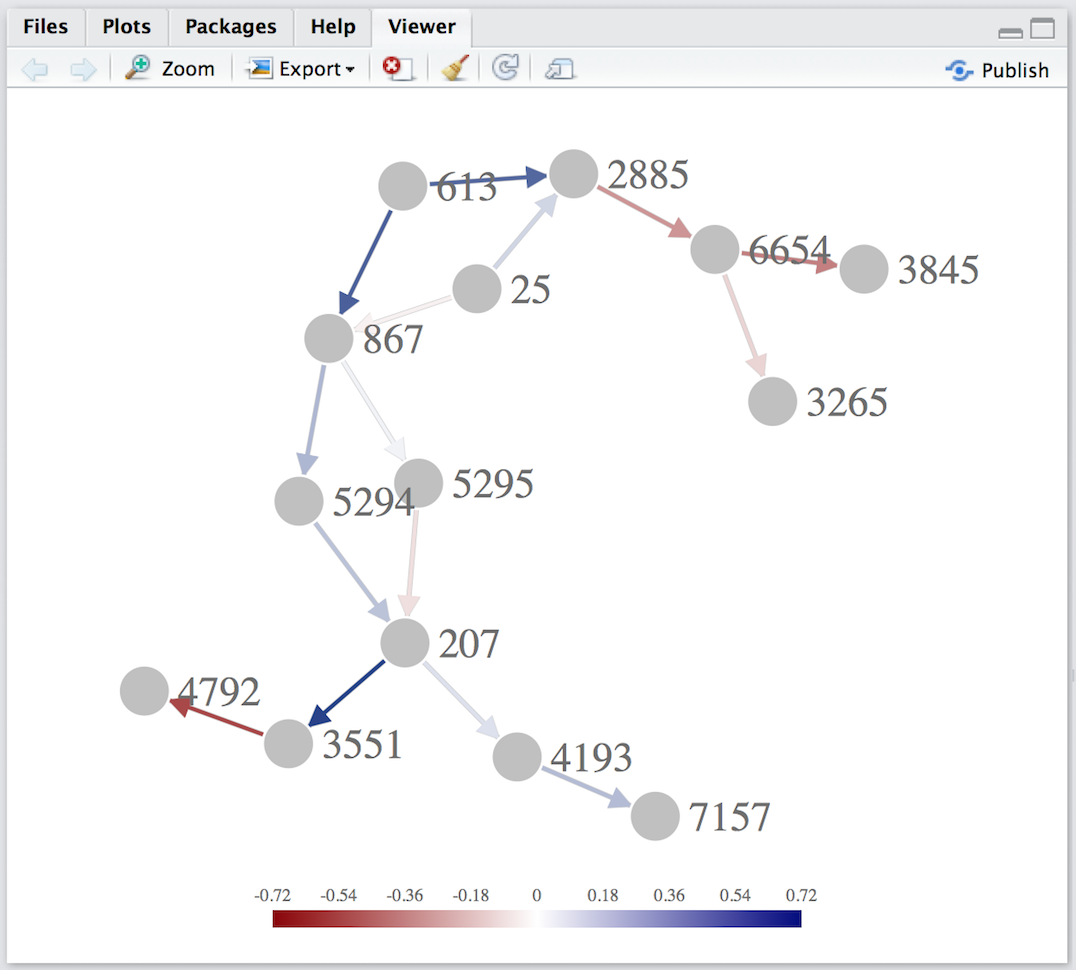# Data preparation

We consider the acute lymphocytic leukemia dataset, included with the simPATHy package, first published in (Chiaretti et al. 2005). This dataset contains expression of 3405 genes in two conditions with sample sizes \(n_1=37\) and \(n_2=41\).

``````library(simPATHy)
library(graph)``````
``````data("chimera")
dim(chimera)``````
``##  3405   78``
``table(colnames(chimera))``
``````##
##  1  2
## 37 41``````

The column names indicate the condition (1 or 2) of each sample.

In this example, we restrict our attention to a subset of genes in this dataset, corresponding to genes participating in the KEGG’s ``Acute Myeloid Leukemia’’ pathway.

The graph that we consider in what follows is a directed acyclic graph derived manually from this pathway.

``````graph<-gRbase::dag(~867:25+867:613+5295:867+5294:867+
207:5295+207:5294+4193:207+3551:207+
4792:3551+7157:4193+3265:6654+
3845:6654+6654:2885+2885:25+2885:613)``````

We take the first condition of this dataset as a reference condition for our example and begin by estimating the covariance matrix.

``````genes<-graph::nodes(graph)
data<-t(chimera[genes,colnames(chimera)==1])
S<-cov(data) ``````

The matrix `S` does not reflect conditional independence constraints imposed by the `graph`. To impose these structural constraints simPATHy provides a function `fitSgraph` for maximum likelihood estimation of covariance matrices in graphical models, for both Gaussian bayesian networks and Gaussian graphical models.

``````S<-fitSgraph(graph,S)
round(S[1:5,1:5],3)``````
``````##         867     25   613  5295   5294
## 867   0.115 -0.006 0.071 0.008  0.045
## 25   -0.006  0.240 0.000 0.000 -0.003
## 613   0.071  0.000 0.121 0.005  0.028
## 5295  0.008  0.000 0.005 0.317  0.003
## 5294  0.045 -0.003 0.028 0.003  0.246``````

The package also provides two plotting functions for graphical models. The first `plotGraphNELD3`, focuses on the graphical structure, while the second one, `plotCorGraph`, focuses on the correlation matrix. In both cases, the colors represent the strength of relation between nodes, where the user by setting the parameter `type` chooses whether to show the pairwise correlation coefficient (`type= "cor"`) or the partial correlation coefficient (`type= "pcor"`).

``plotGraphNELD3(graph,type = "cor",S1 = S)````plotCorGraph(S1 = S,type = "cor")``